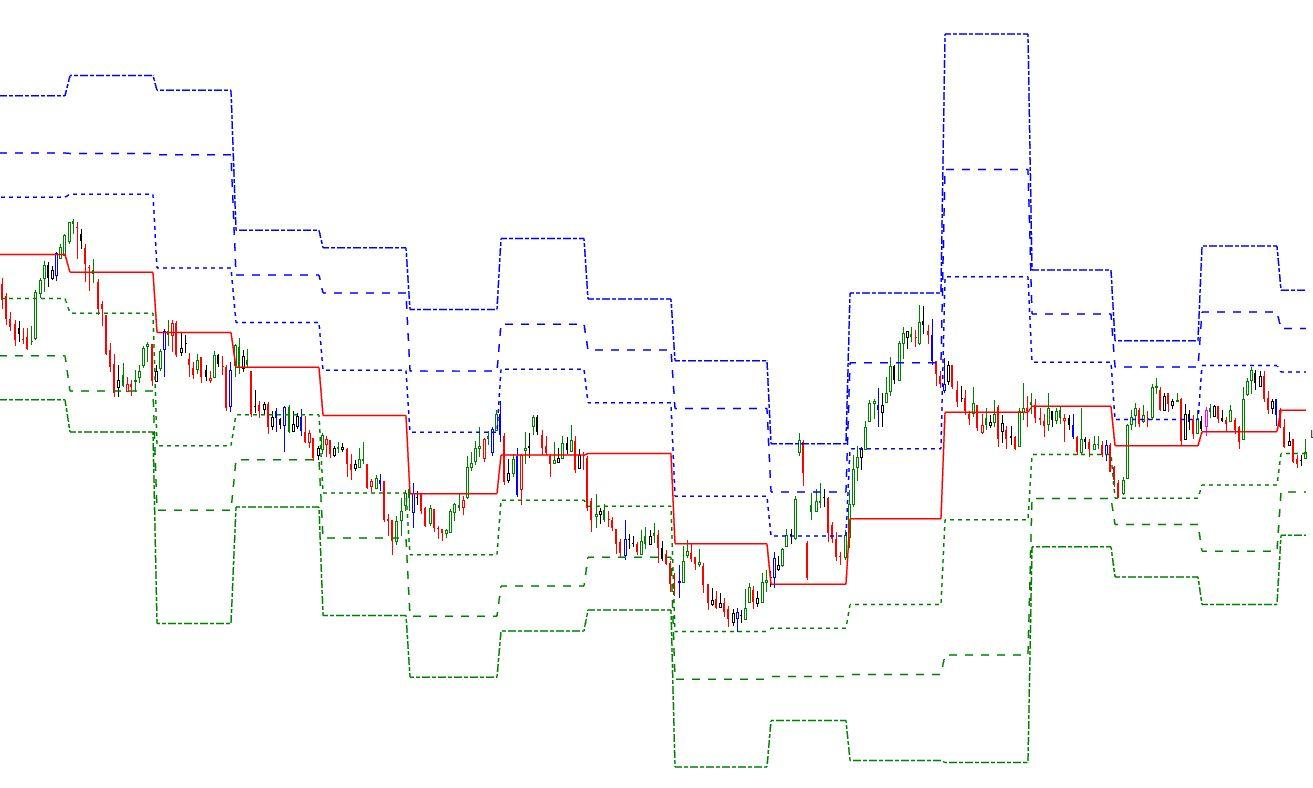Select Page

# Reply To: Drawing pivots using a script

Optuma Forums Optuma Scripting Drawing pivots using a script Reply To: Drawing pivots using a script

#51583

Hi Steve,

I’m guessing that this is what you were hoping to see:The first issue with your scripts is that you’ve assigned variable codes, R1, R2, R3 S1, etc that are the same as parameters used in the PIVOTS() function, eg PIVOTS(DEFAULT=R1, PIVOTS=Monthly) . This will cause the Function to try and call that variable of the same name – a No No! Thus I’ve started all those variables with a “v” to distinguish them from the function parameters.

Next you need to define the Plots you wish to display. So here is the script code that I used to generate the above chart with SHOWPLOT tool calling the script:

// Steve Script – Script Forum #51575 20190312

PP = pivots(PIVOTS=Monthly);

vR1 = pivots(DEFAULT=R1, PIVOTS=Monthly);

vR2 = Pivots(DEFAULT=R2, PIVOTS=Monthly);

vR3 = pivots(DEFAULT=R3, PIVOTS=Monthly);

vS1 = pivots(DEFAULT=S1, PIVOTS=Monthly);

vS2 = pivots(DEFAULT=S2, PIVOTS=Monthly);

vS3 = pivots(DEFAULT=S3, PIVOTS=Monthly);

Plot1 = PP; Plot1.Colour = Red;

Plot1.LineStyle = Solid;

Plot2 = vR1;

Plot2.Colour = Blue;

Plot2.LineStyle = Dash;

Plot3 = vR2;

Plot3.Colour = Blue;

Plot3.LineStyle = LongDash;

Plot4= vR3;

Plot4.Colour = Blue;

Plot4.LineStyle = DashDot;

Plot5 = vS1;

Plot5.Colour = Green;

Plot5.LineStyle = Dash;

Plot6 = vS2;

Plot6.Colour = Green;

Plot6.LineStyle = LongDash;

Plot7= vS3;

Plot7.Colour = Green;

Plot7.LineStyle = DashDot;

Incidentally, if you are wanting a result to use with a SHOWBAR, the final result of your script must not have a “;”, all other lines in the script must have one.

Cheers

Trevor

The Auld Tyma at